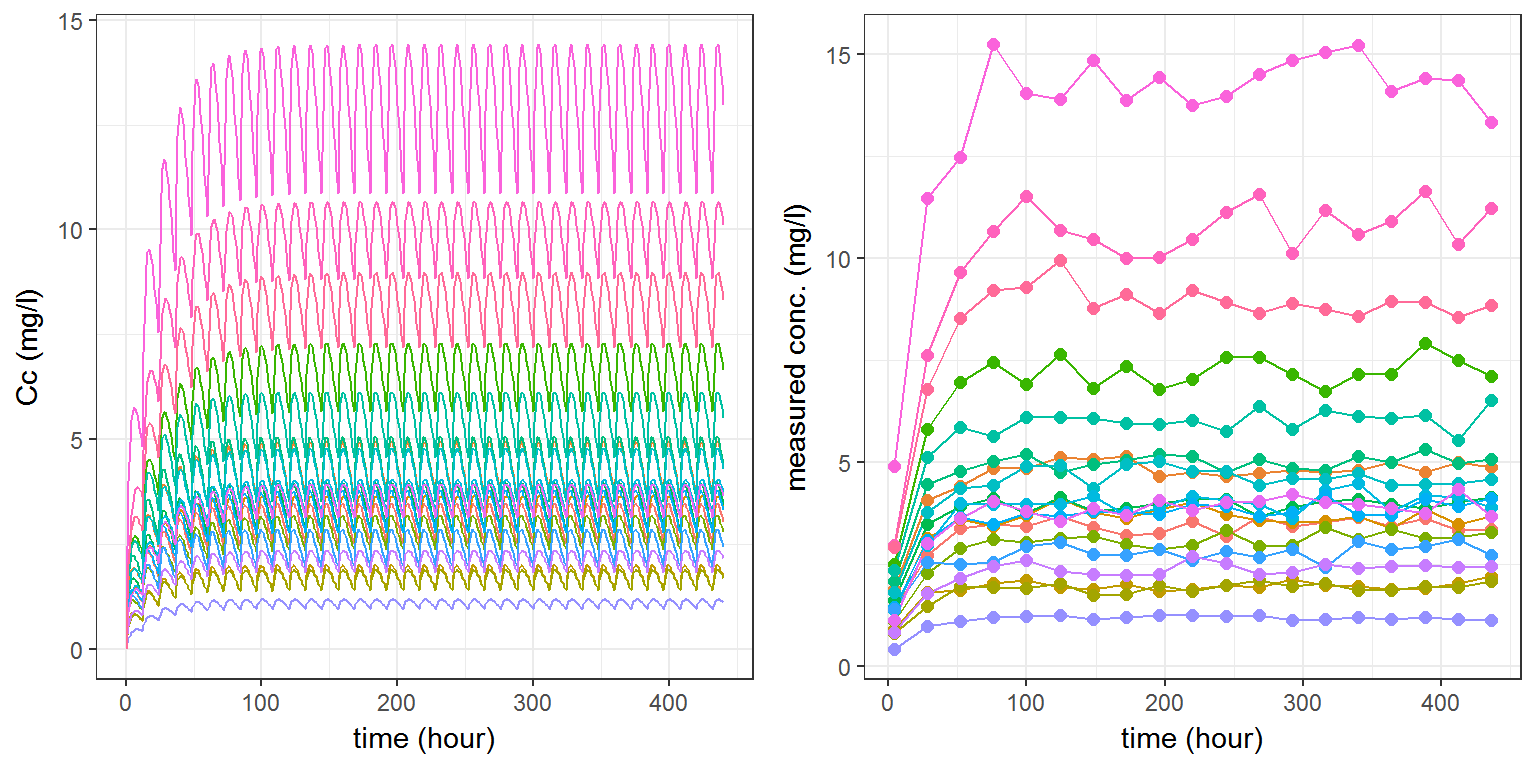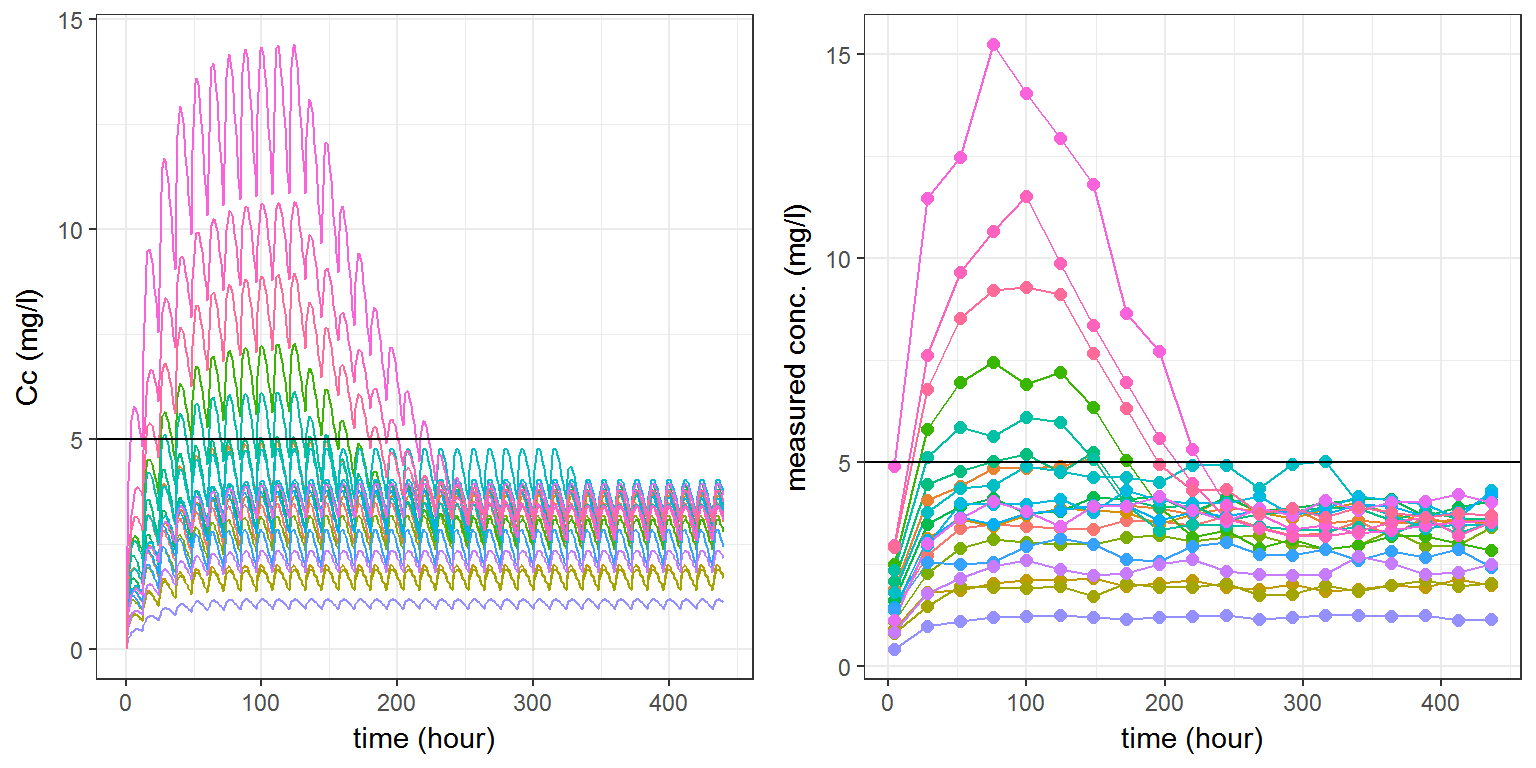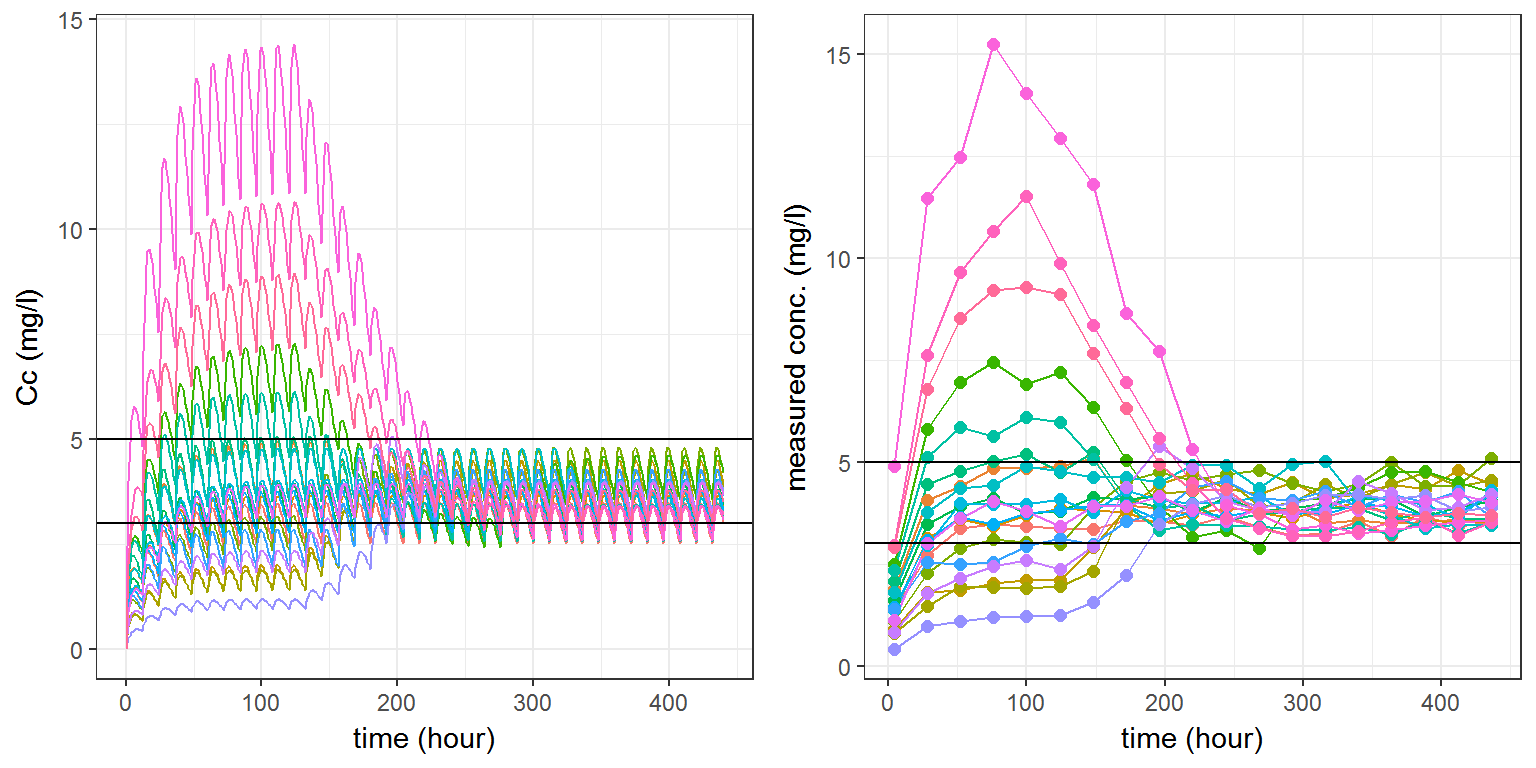$$\newcommand{\esp}{\mathbb{E}\left(#1\right)} \newcommand{\var}{\mbox{Var}\left(#1\right)} \newcommand{\deriv}{\dot{#1}(t)} \newcommand{\prob}{ \mathbb{P}\!(#1)} \newcommand{\eqdef}{\mathop{=}\limits^{\mathrm{def}}} \newcommand{\by}{\boldsymbol{y}} \newcommand{\bc}{\boldsymbol{c}} \newcommand{\bpsi}{\boldsymbol{\psi}} \def\pmacro{\texttt{p}} \def\like{{\cal L}} \def\llike{{\cal LL}} \def\logit{{\rm logit}} \def\probit{{\rm probit}} \def\one{{\rm 1\!I}} \def\iid{\mathop{\sim}_{\rm i.i.d.}} \def\simh0{\mathop{\sim}_{H_0}} \def\df{\texttt{df}} \def\res{e} \def\xomega{x} \newcommand{\argmin}{{\rm arg}\min_{#1}} \newcommand{\argmax}{{\rm arg}\max_{#1}} \newcommand{\Rset}{\mbox{\mathbb{R}}} \def\param{\theta} \def\setparam{\Theta} \def\xnew{x_{\rm new}} \def\fnew{f_{\rm new}} \def\ynew{y_{\rm new}} \def\nnew{n_{\rm new}} \def\enew{e_{\rm new}} \def\Xnew{X_{\rm new}} \def\hfnew{\widehat{\fnew}} \def\degree{m} \def\nbeta{d} \newcommand{\limite}{\mathop{\longrightarrow}\limits_{#1}} \def\ka{k{a}} \def\ska{k{\scriptscriptstyle a}} \def\kel{k{e}} \def\skel{k{\scriptscriptstyle e}} \def\cl{C{\small l}} \def\Tlag{T\hspace{-0.1em}{lag}} \def\sTlag{T\hspace{-0.07em}{\scriptscriptstyle lag}} \def\Tk{T\hspace{-0.1em}{k0}} \def\sTk{T\hspace{-0.07em}{\scriptscriptstyle k0}} \def\thalf{t{1/2}} \newcommand{\Dphi}{\partial_\pphi #1} \def\asigma{a} \def\pphi{\psi} \newcommand{\stheta}{{\theta^\star}} \newcommand{\htheta}{{\widehat{\theta}}}$$

# Task

Individualised therapies are increasingly important for drug development. One reason for individualised therapies is concentration related toxicity and high between-patient variability of exposure levels. In such a case each patient can be monitored for the concentration levels and the drug dose will be adjusted when the individual concentration level exceeds a certain threshold. In this example we demonstrate how Simulx is used to run clinical trial simulations with an adaptive dosing. We will simulate a PK/PD model with a decision rule to adjust the dose based on monitored concentration levels. In addition, we will include a decision rule for the efficacy as well to ensure that desired efficacy level will be maintained.

# The PK model

Tne PK model is a one compartment model for oral administration with first order absorption and elimination.

Parameters $$ka$$, $$V$$ and $$k$$ are log-normally distributed

This PK model is implemented in a text file cts1a.txt:

[LONGITUDINAL]
input = {ka, V, k, a1}
EQUATION:
Cc = pkmodel(ka, V, k)
DEFINITION:
y1 = {distribution=lognormal, prediction=Cc, sd=a1}

[INDIVIDUAL]
input={ka_pop, omega_ka, V_pop, omega_V, k_pop, omega_k}
DEFINITION:
ka = {distribution=lognormal, prediction=ka_pop, sd=omega_ka}
V  = {distribution=lognormal, prediction=V_pop,  sd=omega_V}
k  = {distribution=lognormal, prediction=k_pop,  sd=omega_k}


# Simulation of PK data

We can use simulx for simulating a clinical trial with 20 patients to whom a dose of 20mg is administrated orally every 12 hours starting at time 0.

We then want to compute for these 20 patients:

• the predicted concentration $$Cc$$ every hour between times 0h and 440h
• the observed concentration $$y_1$$ every 24 hours between time 4h and 440h

adm <- list(time=seq(0,to=440,by=12), amount=20)

ppk <- c(ka_pop=0.4,   V_pop=10,    k_pop=0.05,
omega_ka=0.3, omega_V=0.5, omega_k=0.1,
a1=0.05)

g   <- list(size=20, level='individual')

Cc <- list(name='Cc', time=seq(0,to=440,by=1))
y1  <- list(name='y1', time=seq(4,to=440,by=24))

s   <- list(seed=1234)

res1 <- simulx(model     = "model/cts1a.txt",
parameter = ppk,
treatment = adm,
group     = g,
output    = list(Cc, y1),
settings  = s)

(we use seed = 1234 for reproducibility reason. Different results will be obtained with different values of the seed.)

We can now plot the predicted and observed concentrations:

library("gridExtra")
library(ggplot2)
theme_set(theme_bw())
plot1 <- ggplot(data=res1$Cc, aes(x=time, y=Cc, colour=id)) + geom_line(size=0.5) + xlab("time (hour)") + ylab("Cc (mg/l)") + theme(legend.position="none") plot2 <- ggplot(data=res1$y1, aes(x=time, y=y1, colour=id)) +
geom_line(size=0.5) + geom_point(size=2) +
xlab("time (hour)") + ylab("measured conc. (mg/l)") +
theme(legend.position="none")
grid.arrange(plot1, plot2, ncol=2)# Introducing a single decision rule

Assume now that, for safety reason, the concentration should remain below a given threshold of 5 mg/l.

The exposure, i.e. the true’’ concentration $$Cc$$, is above this threshold for several patients. Since $$Cc$$ cannot be measured in practice, we can then decide to reduce the dose for those patients which measured concentration is above 5 mg/l.

We thus define an adaptive individual design as follows:

• Visits are scheduled every 24 hours, starting at time 124h.
• If, at a given visit, the observed concentration $$y_1$$ of a patient is greater than 5, then the next doses administrated to this patient will be reduced, by multiplying the current amount by a factor 0.75.

This decision rule is implemented as a R list:

r1 <- list(name='y1',
time=seq(124,to=440,by=24),
condition="y1>5",
factor=0.75)

R function titration is a variant of simulx which now takes this rule into account.

Remark: function titration.R is not part of the mlxR package. This is just an example of what can be done: this function can be freely used/modified by anyone.

source("titration.R")
res2 <- titration(model     = "model/cts1a.txt",
parameter = ppk,
treatment = adm,
output    = list(Cc, y1),
rule      = r1,
group     = g,
settings  = s)

Outputs of titration and simulx have similar structure. We can therefore display the predicted and observed concentrations obtained with titration as we did previously with the output of simulx:

plot3 <- ggplot(data=res2$Cc, aes(x=time, y=Cc, colour=id)) + geom_line(size=0.5) + xlab("time (hour)") + ylab("Cc (mg/l)") + theme(legend.position="none") + geom_hline(yintercept=5) plot4 <- ggplot(data=res2$y1, aes(x=time, y=y1, colour=id))     +
geom_line(size=0.5) + geom_point(size=2) +
xlab("time (hour)") + ylab("measured conc. (mg/l)")           +
theme(legend.position="none") + geom_hline(yintercept=5)
grid.arrange(plot3, plot4, ncol=2)What was expected is now clearly observed: high concentrations tend to reduce after the first visit at time 124.

# Combining several decision rules

We can introduce several decision rules. Imagine for instance that efficacy requires a concentration above 3 mg/l. We can then decide, for example, to multiply the dose by a factor 1.5 when the observed concentration is below this value at a given visit.

We can then implement this new decision rule as a new list:

r2 <- list(name='y1',
time=seq(124,to=440,by=24),
condition="y1<3",
factor=1.5)

We will now use titration combining the two decisions rules r1 and r2:

res3 <- titration(model     = "model/cts1a.txt",
parameter = ppk,
treatment = adm,
output    = list(Cc, y1),
rule      = list(r1,r2),
group     = g,
settings  = s)

plot5 <- ggplot(data=res3$Cc, aes(x=time, y=Cc, colour=id)) + geom_line(size=0.5) + xlab("time (hour)") + ylab("Cc (mg/l)") + theme(legend.position="none") + geom_hline(yintercept=c(3,5)) plot6 <- ggplot(data=res3$y1, aes(x=time, y=y1, colour=id))     +
geom_line(size=0.5) + geom_point(size=2) +
xlab("time (hour)") + ylab("measured conc. (mg/l)")   +
theme(legend.position="none") + geom_hline(yintercept=c(3,5))
grid.arrange(plot5, plot6, ncol=2)# Extension to PKPD data

Assume now that we have PK and PD data. In this example, the PD model is a Emax model.

The joint PKPD model is implemented in a new file cts1b.txt:

[LONGITUDINAL]
input = {ka, V, k, Emax, EC50, a1, a2}
EQUATION:
Cc = pkmodel(ka, V, k)
E  = Emax*Cc/(EC50+Cc)

DEFINITION:
y1 = {distribution=lognormal, prediction=Cc, sd=a1}
y2 = {distribution=normal, prediction=E,  sd=a2}

[INDIVIDUAL]
input={
ka_pop, omega_ka, V_pop, omega_V, k_pop, omega_k,
Emax_pop, omega_Emax, EC50_pop, omega_EC50
}
DEFINITION:
ka   = {distribution=lognormal, prediction=ka_pop,   sd=omega_ka}
V    = {distribution=lognormal, prediction=V_pop,    sd=omega_V}
k    = {distribution=lognormal, prediction=k_pop,    sd=omega_k}
Emax = {distribution=lognormal, prediction=Emax_pop, sd=omega_Emax}
EC50 = {distribution=lognormal, prediction=EC50_pop, sd=omega_EC50}

We need to provide the values of the population PD parameters for simulating PKPD data from this model.

ppd <- c(Emax_pop=100,   EC50_pop=3,
omega_Emax=0.1, omega_EC50=0.2, a2=5)

E <-  list(name='E',  time=seq(0,to=440,by=1))
y2 <- list(name='y2', time=seq(16,to=440,by=36))

res4 <- simulx(model     = "model/cts1b.txt",
parameter = c(ppk,ppd),
treatment = adm,
output    = list(Cc, E, y1, y2),
group     = g,
settings  = s)

We can now plot the predicted and observed PK and PD data for the 20 patients:

pl1=ggplot(data=res4$Cc, aes(x=time, y=Cc, colour=id))+geom_line(size=0.5)+ xlab("time (hour)") + ylab("Cc (mg/l)") + theme(legend.position="none") pl2=ggplot(data=res4$E, aes(x=time, y=E, colour=id))+geom_line(size=0.5)  +
xlab("time (hour)") + ylab("E") + theme(legend.position="none")
pl3=ggplot(data=res4$y1, aes(x=time, y=y1, colour=id))+geom_line(size=0.5)+ geom_point(size=2) + xlab("time (hour)") + ylab("measured conc (mg/l)") + theme(legend.position="none") pl4=ggplot(data=res4$y2, aes(x=time, y=y2, colour=id))+geom_line(size=0.5)+
geom_point(size=2) + xlab("time (hour)") + ylab("measured effect")      +
theme(legend.position="none")

grid.arrange(pl1, pl2, pl3, pl4, ncol=2)